International
Tables for
Crystallography
Volume H
Powder diffraction
Edited by C. J. Gilmore, J. A. Kaduk and H. Schenk

International Tables for Crystallography (2018). Vol. H, ch. 3.8, pp. 327-328

## Section 3.8.3.2. Estimating the number of clusters

C. J. Gilmore,a G. Barra and W. Donga*

aDepartment of Chemistry, University of Glasgow, University Avenue, Glasgow, G12 8QQ, UK
Correspondence e-mail:  chris@chem.gla.ac.uk

#### 3.8.3.2. Estimating the number of clusters

| top | pdf |

An estimate of the number of clusters present in the data set is needed. In terms of the dendrogram, this is equivalent to `cutting the dendrogram' i.e. the placement of a horizontal line across it such that all the clusters as defined by tie lines above this line remain independent and unlinked. The estimation of the number of clusters is an unsolved problem in classification methods. It is easy to see why: the problem depends on how similar the patterns need to be in order to be classed as the same, and how much variability is allowed within a cluster. We use two approaches: (a) eigenvalue analysis of matrices ρ and A, and (b) those based on cluster analysis.

Eigenvalue analysis is a well used technique: the eigenvalues of the relevant matrix are sorted in descending order and when a fixed percentage (typically 95%) of the data variability has been accounted for, the number of eigenvalues is selected. This is shown graphically via a scree plot, an example of which is shown in Fig. 3.8.2.Figure 3.8.2 | top | pdf |Four different methods of estimating the number of clusters present in a set of 23 powder patterns for the drug doxazosin. A total of five polymorphs are present, as well as two mixtures of these polymorphs. (a) A scree plot from the eigenvalue analysis of the correlation matrix; (b) the use of the C test (the coefficients have been multiplied by 100.0), which gives an estimate of five clusters using its local maximum. The γ test estimates that there are seven clusters and the CH test has a local maximum at seven clusters. Numerical details are given in Table 3.8.2.

We carry out eigenvalue analysis on the following:

 (1) Matrix ρ. (2) Matrix A, as described in Section 3.8.3.3. (3) A transformed form of ρ in which ρ is standardized to give ρs in which the rows and columns have zero mean and unit variance. The matrix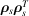is then computed and subjected to eigenanalysis. It tends to give a lower estimate of cluster numbers than (1).

The most detailed study on cluster counting is that of Milligan & Cooper (1985), and is summarized by Gordon (1999). From this we have selected three tests that seem to operate effectively with powder data:

 (4) The Calinški & Harabasz (1974) (CH) test: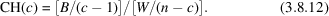A centroid is defined for each cluster. W denotes the total within-cluster sum of squared distances about the cluster centroids, and B is the total between-cluster sum of squared distances. Parameter c is the number of clusters chosen to maximize CH. (5) A variant of Goodman & Kruskal's (1954) γ test, as described by Gordon (1999). The dissimilarity matrix is used. A comparison is made between all the within-cluster dissimilarities and all the between-cluster dissimilarities. Such a comparison is marked as concordant if the within-cluster dissimilarity is less than the between-cluster dissimilarity, and discrepant otherwise. Equalities, which are unusual, are disregarded. If S+ is the number of concordant and S− the number of discrepant comparisons, then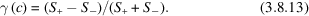A maximum in γ is sought by an appropriate choice of cluster numbers. (6) The C test (Milligan & Cooper, 1985). This chooses the value of c that minimizes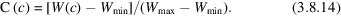W(c) is the sum of all the within-cluster dissimilarities. If the partition has a total of r such dissimilarities, then Wmin is the sum of the r smallest dissimilarities and Wmax is the sum of the r largest.

The results of tests (4)–(6) depend on the clustering method being used. To reduce the bias towards a given dendrogram method, these tests are carried out on four different clustering methods: the single-link, the group-average, the sum-of-squares and the complete-link methods. Thus there are 12 semi-independent estimates of the number of clusters from clustering methods, and three from eigenanalysis, making 15 in all.

A composite algorithm is used to combine these estimates. The maximum and minimum values of the number of clusters (cmax and cmin, respectively) given by the eigenanalysis results [(1)–(3) above] define the primary search range; tests (4)–(6) are then used in the range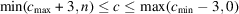to find local maxima or minima as appropriate. The results are averaged, any outliers are removed, and a weighted mean value is taken of the remaining indicators, then this is used as the final estimate of the number of clusters. Confidence levels for c are also defined by the estimates of the maximum and minimum cluster numbers after any outliers have been removed.

A typical set of results for the PXRD data from 23 powder patterns for doxazosin (an anti-hypertension drug) in which five polymorphs are present, as well as two mixtures of polymorphs, is shown in Fig. 3.8.2(a) and (b) (see also Table 3.8.2). The scree plot arising from the eigenanalysis of the correlation matrix indicates that 95% of the variability can be accounted for by five components, and this is shown in Fig. 3.8.2(a). Eigenvalues from other matrices indicate that four clusters are appropriate. A search for local optima in the CH, γ and C tests is then initiated in the range 2–8 possible clusters. Four different clustering methods are tried, and the results indicate a range of 4–7 clusters. There are no outliers, and the final weighted mean value of 5 is calculated. As Fig. 3.8.2(b) shows, the optimum points for the C and γ tests are often quite weakly defined (Barr et al., 2004b).

 Table 3.8.2| top | pdf | Estimate of the number of clusters for the 23 sample data set for doxazosin
 There are five polymorphs present, plus two mixtures of these polymorphs. The maximum estimate is 7; the minimum estimate is 4; the combined weighted estimate of the number of clusters is 6, and the median value is 5. The dendrogram cut level is set to give 5 clusters, and the lower and upper confidence limits are 4 and 7, respectively.
MethodNo. of clusters
Principal-component analysis (non-transformed matrix) 5
Principal-component analysis (transformed matrix) 4
Multidimensional metric scaling 4
γ statistic using single linkage 7
CH statistic using single linkage 7
C statistic using single linkage
γ statistic using group averages 7
CH statistic using group averages 5
C statistic using group averages
γ statistic using sum of squares
CH statistic using sum of squares 5
C statistic using sum of squares
γ statistic using complete linkage
CH statistic using complete linkage 5
C statistic using complete linkage

### References

Barr, G., Dong, W. & Gilmore, C. J. (2004a). High-throughput powder diffraction. II. Applications of clustering methods and multivariate data analysis. J. Appl. Cryst. 37, 243–252.Google Scholar
Calinški, T. & Harabasz, J. (1974). A dendritic method for cluster analysis. Commun. Stat. 3, 1–27.Google Scholar
Goodman, L. A. & Kruskal, W. H. (1954). Measures of association for cross-classifications. J. Am. Stat. Assoc. 49, 732–764.Google Scholar
Gordon, A. D. (1999). Classification, 2nd ed. Boca Raton: Chapman and Hall/CRC.Google Scholar
Milligan, G. W. & Cooper, M. C. (1985). An examination of procedures for determining the number of clusters in a data set. Psychometrika, 50, 159–179.Google Scholar